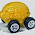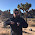## Sunday, March 15, 2015

### Utility in an information equilibrium model

I've said many times on this blog that the concept of utility is unnecessary (except to solve an information problem); in this post I'll allow utility, but show that even then utility maximization is unnecessary to select an economic equilibrium.  Essentially, I will derive the utility approach to economics using the information transfer model.

Let's define utility $U(x_{1}, x_{2}, ...)$ to be the information source in the markets

$$MU_{x_{i}} : U \rightarrow x_{i}$$

for $i = 1 ... D$ where $MU_{x_{i}}$ is the marginal utility (a detector) for the good $x_{i}$ (information destination). We can immediately write down the main information transfer model equation:

$$MU_{x_{i}} = \frac{\partial U}{\partial x_{i}} = k_{i} \; \frac{U}{x_{i}}$$

Solving the differential equations, our utility function $U(x_{1}, x_{2}, ...)$ is

$$U(x_{1}, x_{2}, ...) = a \prod_{i} \left( \frac{x_{i}}{c_{i}} \right)^{k_{i}}$$

which is a Cobb-Douglas utility function ($a$ and the $c_{i}$ are constant). In the traditional economic approach, the next step is to look at the level curves of this utility function alongside the budget constraint (in a two-good market i.e. two dimensions):

$$\text{(1) }\; M \geq \sum_{i} p_{i} x_{i} = p_{1} x_{1} + p_{2} x_{2}$$

The level curve that is tangent to the budget constraint gives us the maximized utility. We show this in the next graph (level curve is the dashed gray curve and the maximized utility point is gray as well):

The entropy maximizing solution is given as the blue dot at the centroid of the blue triangle with the solid utility level curve passing through it. If we take every point that satisfies the budget constraint (1) as equally likely (the blue shaded region), the expected equilibrium value is given by the centroid. Now this centroid moves in effectively the same way as the utility maximum, just at a lower utility value:

The red line shows that at a higher price of $x_{1}$ with the same budget constraint, less of $x_{1}$ can be afforded. Both the utility maxima (gray dots) and the entropy maxima (blue and red dots) move inward towards smaller $x_{1}$ and lower utility, tracing out a demand curve. For two goods, there is only one big difference in the utility maximizing and entropy maximizing models of supply and demand: the entropy maximizing equilibrium doesn't fully saturate the budget constraint.

One possibility is to make the artificial restriction that all income $M$ must be spent. The centroid of the boundary of the blue triangle would essentially be the middle point of the budget constraint line. However, we don't actually have to do this.

If we randomly sample the triangle bounded by the budget constraint, we end up with something like this in two dimensions:

The distribution of the sum $p_{1} x_{1} + p_{2} x_{2}$ is somewhat uniformly distributed between 0 and the budget constraint as shown by the blue line in the next graph:

However, if we increase the number of dimensions (the number of goods in the economy), most of the points are near the budget constraint (the red and green lines). This is a general property of higher dimensional shapes: nearly all of the points are in a thin shell near the surface. That means for a large number of dimensions, the expected equilibrium should be near the budget constraint even without making the artificial restriction above:

Now this point only maximizes utility if every good is in a sense the same -- the budget constraint (1) and the utility function $U$ is symmetric under interchange of $x_{i} \leftrightarrow x_{j}$. Typically, the entropy maximizing point is near the utility maximizing point if neither the utility function nor the budget constraint are wildly asymmetric ($k_{i} \gg k_{j}$ or $p_{i} \gg p_{j}$).

We can't directly observe utility, though. Therefore it is always possible to perform a preference-preserving transformation $f(x_{i}, \alpha_{i}) : x_{i} \rightarrow \exp \alpha_{i} \log x_{i}$ that makes the utility level surface tangent at the maximum entropy point near the budget constraint (or makes the utility function symmetric). [Correction, per LAL in comments below.]

Aside: This framework would allow us to experimentally determine whether utility maximization or entropy maximization represented a better model of microeconomics. Using multiple goods, aggregate the different allocations chosen by individual subjects (every student in a classroom is given $n$ tokens to allocate among $m$ different goods like candy bars or bacon).

Second aside: The typical economics approach appears to be setting $MU_{x_{i}} = \beta p_{i}$; we could accommodate that with an additional information equilibrium condition $MU_{x_{i}} \sim p_{i}$ which would allow $\log MU \simeq k \log p$. The typical approach assumes $k = 1$.

#### 7 comments:

1.fascinating stuff...so the entropy maximizing point is dependent on utility transformations? and the transformation you suggested is there a particular reason to make it? or is it just one that works?

1.Cheers, LAL.

The realized value of utility evaluated at the entropy maximizing point can be changed by making utility transformations. Unlike utility maximizing point, the location of the entropy maximizing point is independent of the utility functions.

The particular form of the transformation I wrote down scales the values of the exponents in the Cobb Douglas utility function at the top of the post. I can adjust those exponents so the utility level curves are tangent at the entropy maximizing point (in a large number of dimensions), making entropy maximization equivalent to utility maximization.

Another way to put this is that the entropy maximizing and utility maximizing points only have to be distinguishable if you can actually measure prices and utility independently. Otherwise, you can re-scale utility to make the entropy maximizing point the utility maximizing point.

Now this could of course be wrong -- entropy maximization says something specific and observable about where the equilibrium should be (near the middle of the budget constraint curve for a large number of items). I personally think that utility isn't really necessary (I use NGDP as the information source in the macro models) -- I'm mostly showing how one would approach it mathematically using information equilibrium.

This is speculation at this point, but I think there may be a "gauge freedom" in Weinstein's utility gauge theory that explains why one is always able do that re-scaling. More to look into ...

2.I guess I am confused by your term "preference-preserving transformation".

In economics that means a monotonic transformation of the utility curves that would change only the ordinal values of the utility function, but not the ranking of consumer bundles. Hence such a transformation wouldn't change the optimal choice.

If I understand you correctly you are saying the transformation you have written down bends the curves to be tangent to the point on the budget constraint nearest the max entropy point?

3.my above comment is potentially ambiguous: any monotonic transformation preserves the ranking of bundles. you mean to be using the gauge theory to be talking about local transformations near the max entropy?

4.I think you are right and I am wrong here -- the transformation I show isn't actually preference preserving across baskets of goods. It actually changes the allocation.

I will have to re-think that particular argument. However, I'm not sure the "preference preserving" aspect is necessary -- if prices are observable, but utility is not, then you can scale utility to conform with the observed equilibrium. The main question is still: do the observed equilibria correspond to maximum entropy or not (if not they can be described as the maximum utility solution for some utility function, and the maximum entropy equilibrium is not a good model).

What I was attempting to say is that if all of the equilibria observed are maximum entropy equilbria, then there exists a utility function that makes them maximum utility solutions as well and both views are correct. If the equilibria aren't MaxEnt, then MaxEnt is not a good model.

5.Another way, if the transformation was actually preference preserving, then there would be no observable difference between MaxEnt equilibria and Max U(x) equilibria -- and I am saying there is an observable difference!

6.Yes, I think your argument still holds. You can find a rational agent (although probably imaginary) that would have this utility function maximized for the max entropy for a large number of goods. As you point out there is no reason for this agent to have the same utility as an aggregate of the underlying agents or of the individual agents for exotic preferences or well behaved preferences over a small number of goods...

However I think with this you could probably prove some pretty strong bounds about the maximum inefficiency (well-fare loss) in choosing the max entropy vs max utility for rational agents with continuous, monotonic, convex preferences.

Comments are welcome. Please see the Moderation and comment policy.

Also, try to avoid the use of dollar signs as they interfere with my setup of mathjax. I left it set up that way because I think this is funny for an economics blog. You can use € or £ instead.